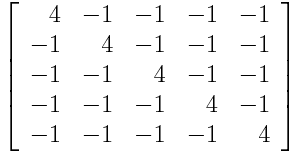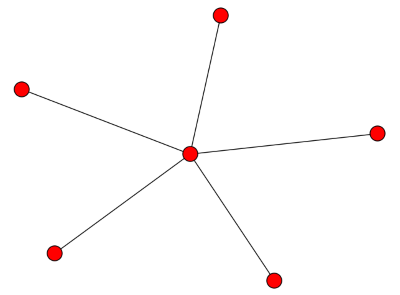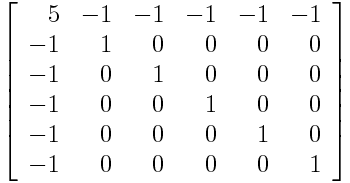# Laplacian Spectrum of Complete Graphs, Stars, and Rings

### Here's a little extra math to add to your understanding of graph theory.

· Big Data Zone · Analysis
Save
3.49K Views

a few examples help build intuition for what the eigenvalues of the graph laplacian tell us about a graph. the smallest eigenvalue is always zero (see explanation in footnote here ).

for a complete graph on n vertices, all the eigenvalues except the first equal n . the eigenvalues of the laplacian of a graph with n vertices are always less than or equal to n , this says the complete graph has the largest possible eigenvalue. this makes sense in light of the previous post: adding edges increases the eigenvalues . when you add as many edges as possible, you get the largest possible eigenvalues.next we look at the case of a star with n -1 vertices connected to a central vertex. the smallest eigenvalue is zero, as always, and the largest is n . the ones in the middle are all 1. in particular, the second eigenvalue , the algebraic connectivity, is 1. it’s positive because the graph is connected, but it’s not large because the graph is not well connected: if you remove the central vertex it becomes completely disconnected.finally, we look at a cyclical graph, a ring with n vertices. here the eigenvalues are 2 – 2 cos(2π k / n ) where 0 ≤ k n/ 2. in particular, smallest non-zero eigenvalue is 2 – 2 cos(2π/ n ) and so as n increases, the algebraic connectivity approaches zero. this is curious since the topology doesn’t change at all. but from a graph theory perspective, a big ring is less connected than a small ring. in a big ring, each vertex is connected to a small proportion of the vertices, and the average distance between vertices is larger.

Topics:
graph, algorithm, math

Published at DZone with permission of John Cook, DZone MVB.

Opinions expressed by DZone contributors are their own.